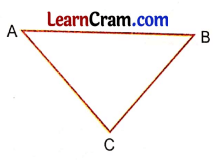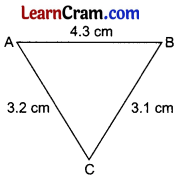# DAV Class 5 Maths Chapter 16 Worksheet 3 Solutions

The DAV Class 5 Maths Solutions and DAV Class 5 Maths Chapter 16 Worksheet 3 Solutions of Triangles offer comprehensive answers to textbook questions.

## DAV Class 5 Maths Ch 16 Worksheet 3 Solutions

Question 1.
Find whether it is possible to draw a triangle with line segments of lengths given below:
(a) 3 cm, 4 cm, 7 cm
Solution:
3 cm, 4 cm, 7 cm
Sum of two side = 3 cm + 4 cm = 7 cm
Third side = 7 cm
So, 7 cm = 7 cm
The sum of two sides should be greater than the third side.
Not possible.(b) 6 cm, 8 cm, 7 cm
Solution:
6 cm, 8 cm, 7 cm
Sum of 2 sides = 6 cm + 8 cm = 14 cm
Third side = 7 cm
So 14 > 7 possible
Sum of 2 sides = 8 cm + 7 cm = 15 cm
Third side = 6 cm
15 cm > 6 cm possible
or
Sum of 2 side = 6 cm + 7 cm = 13 cm
Third side = 8 cm
13 cm > 8 cm possible
So triangle is possible.

(c) 12 cm, 12 cm, 12 cm
Solution:
12 cm, 12 cm, 12 cm
Sum of two side = 12 cm + 12 cm = 24 cm
Third side = 12 cm
24 cm > 12 cm possible.
All the sides are equal so the result is the same in either case.
So to draw a triangle is possible.

(d) 4 cm, 4 cm, 9 cm
Solution:
4 cm, 4 cm, 9 cm
Sum of two side = 4 cm + 4 cm = 8 cm
Third side = 9 cm
8 cm < 9 cm
So, not possible.Question 2.
Measure the sides of the triangle given below and verify that the sum of the length of any two sides of a triangle is greater than the length of its third side.Solution:
In ∆ABCAB = 4.3 cm
AC = 3.2 cm
BC = 3.1 cm
Let us add the sides in pairsAll the conditions are verified.